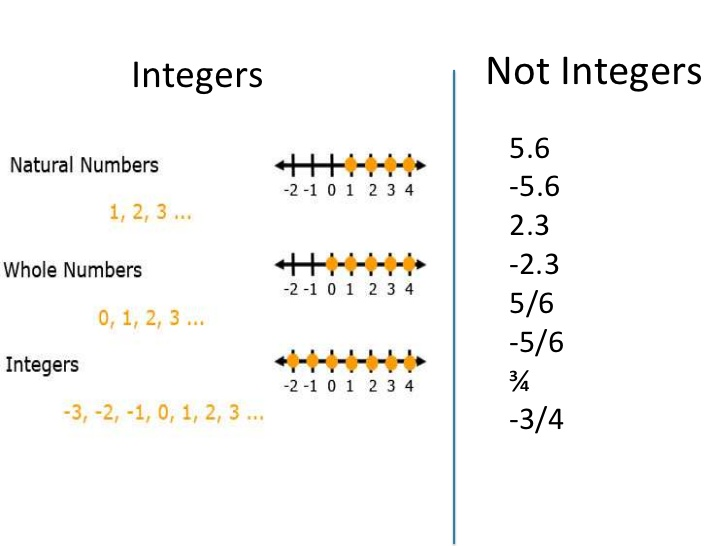# Basic Operation of Integers

Welcome to JSS2!

In today’s class, We will be discussing Basic Operation of Integers. We hope you enjoy the class!

• Definition
• Indices
• Laws of Indices

### Definition of Integer

An integer is any positive or negative whole numberExample:

Simplify the following

I.  (+8) + (+3)           ii. (+9) –  (+4)

Solution

I.   (+8) + (+3) = +11                 ii   (+9) – (+4) = 9-4 = +5 or 5

Self Evaluation

Simplify the following

i.  (+12) –(+7)              ii. 7-(-3)-(-2)### Indices

The plural of index is indices

10 x 10 x 10= 103 in index form, where 3 is the index or power of 10.

P5 =p x p x p x p x p. 5 is the power or index of p in the expression P5.##### Laws of Indices
• Multiplication law:

ax x ay = ax+y

E.g. a5xa3 = a x a x a x a x a x a x a x a =a8

y1 x y4 = y 1+4  = y5

ax a5 = a3 + 5 = a8

4c4 x 3c2

= 4 x 3 x c4 x c2 = 12 x c4+2 =12c6

Classwork

Simplify the following

(a) 103 x 104          (b) 3 x 106 x 4 x 102     (c) p3 x p       (d) 4f3  x  5f

• Division law

(1)  ax ÷ ay = ax ÷ ay = ax-y

Example

Simplify the following

(1)  a7÷a3=a x a x a x a x a x a x a ÷ a x a x a

a7-3 = a4

(2) 106÷103=106÷103=106-3=103

(3) 10a7÷2a2=10a7÷2a2=5a7-2=5a5

Classwork

Simplify the following

A. 105÷103        B.  51m9÷3m            C. 8×109÷4×106

• By division law

ax-x=a0

a0=1

E.g. 1000 =1

500=1

• Zero indexes

ax ÷ ax =

• Negative index

a0 ÷ ax = 1/ax

But by division law, a0-x=a-x

Therefore, a-x=1/ax

Example

1. Simplify (i) b-2 (ii) 2-3

Solution

b-2 = 1/ b2                           (ii) 2-3 = 1/2= 1/2x2x2 = 1/8

Class work

(1) 10-2     (2) d0 x d4 x d-2          (3) a-3÷a-5      (4)  (1/4)-2

• Power of index

[am]n = amxn ${a}^{mn}$

E.g. [a2]4= x a2 x a2 x a2 = a x a x a x a x a x a x a x a=a8

Therefore. a2×4=a8.

(6)   [mn] a=m ax na = mana. e.g. [4+2x] 2 = 42+22xx2  =

16+4x2 = 4[4+1xx2] = 4[4+x2].

•       Fractional indexes

am/n   = a1/n xm= n√ am

Example

1. (a1/2)2 = a2/2 = a1 =a
2. (√a)2 = √a x √a = √a x a = √a2 = a      e.g. 321/55√321
3. 323/5  = 5√32= 5√25×3 = 23 = 2x2x2 = 8
4. 272/3 = 3√272 = 32 = 3x3x3 = 9
5. 4-3/2 = √(1/4)3= 1/23
6. (0001)3 =1×10-3  = (10-3)3=10-3×3=10-9 = $\frac{1}{1000000000}$  =0.000000001
7. (am)p/q = amp = √(a)p
8. (162)3/4 = √ (162)3 = (22)3 = (4)3=4x4x4 = 64

• The Equator of power for equal base

Ax =Ay , That is x = yNew General Mathematics, UBE Edition, chapter 2 Pages 24-26

Essential Mathematics by A J S Oluwasanmi, Chapter 3 pages 27-29

WEEKEND ASSIGNMENT

1. Simplify (+13) – (+6)

(a)7  (b) -7   (c) 19           (d) 8

1. Simplify (+11) – (+6) – (-3)

(a)7 (b)8    (c)9   (d)10

1. Simplify 5x3 x 4x7 (a) 20x4 (b) 20x10 (c) 20x7         (d) 57x10
2. Simplify 10a8 ÷ 5a6 (a) 2a2 (b) 50a2           (c) 2a14           (d) 2a48
3. Simplify r7 ÷ r7 (a) 0 (b) 1   (c) r14  (d) 2r7

THEORY

1. Simplify
• 5y5 x 3y3
• 24 x 8
• 6x
1. Simplify (1/2)-3

We have come to the end of this class. We do hope you enjoyed the class?

Should you have any further question, feel free to ask in the comment section below and trust us to respond as soon as possible.

In our next class, we will be talking about Whole Numbers and Decimal Numbers. We are eager to meet you there.

For more class notes, videos, homework help etc download our Mobile App HERE

Join ClassNotes.ng Telegram Community HERE for exclusive content and support

### 5 thoughts on “Basic Operation of Integers”

1.I love this lesson

2.You are trying

1.Seriously

3.1.